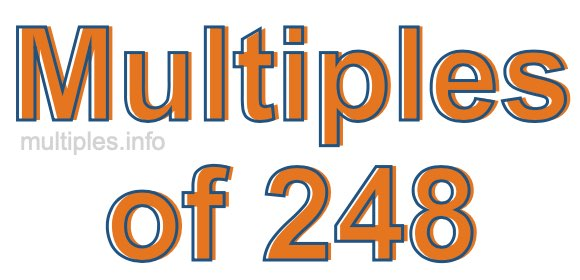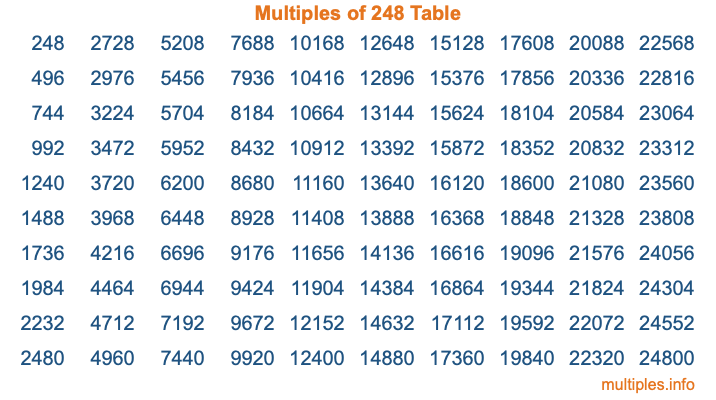Multiples of 248Welcome to the Multiples of 248 page. Here we will first teach you everything you will ever need to know about the multiples of 248, and then give you a study guide summary of everything we taught you to make sure you remember it all. Use this page to look up facts and learn information about the multiples of 248. This page will make you a multiples of two hundred forty-eight expert!

Definition of Multiples of 248
Multiples of 248 are all the numbers that when divided by 248 equal an integer. Each of the multiples of 248 are called a multiple. A multiple of 248 is created by multiplying 248 by an integer.

Therefore, to create a list of multiples of 248, you start with 1 multiplied by 248, then 2 multiplied by 248, then 3 multiplied by 248, and so on for as long as you want. Thus, the list of the first five multiples of 248 is 248, 496, 744, 992, and 1240. To see a larger list of multiples of 248, see the printable image of Multiples of 248 further down on this page. We also have a category where you can choose any nth multiple of 248.

Multiples of 248 Checker
The Multiples of 248 Checker below checks to see if any number of your choice is a multiple of 248. In other words, it checks to see if there is any number (integer) that when multiplied by 248 will equal your number. To do that, we divide your number by 248. If the the quotient is an integer, then your number is a multiple of 248.

Is  a multiple of 248?

Least Common Multiple of 248 and ...
A Least Common Multiple (LCM) is the lowest multiple that two or more numbers have in common. This is also called the smallest common multiple or lowest common multiple and is useful to know when you are adding our subtracting fractions. Enter one or more numbers below (248 is already entered) to find the LCM.

Check out our LCM Calculator if you need more details about the Least Common Multiple or if you need the LCM for different numbers for adding and subtraction fractions.

nth Multiple of 248
As we stated above, 248 is the first multiple of 248, 496 is the second multiple of 248, 744 is the third multiple of 248, and so on. Enter a number below to find the nth multiple of 248.

th multiple of 248

Multiples of 248 vs Factors of 248
248 is a multiple of 248 and a factor of 248, but that is where the similarities end. All postive multiples of 248 are 248 or greater than 248. All positive factors of 248 are 248 or less than 248.

Below is the beginning list of multiples of 248 and the factors of 248 so you can compare:

Multiples of 248: 248, 496, 744, 992, 1240, etc.

Factors of 248: 1, 2, 4, 8, 31, 62, 124, 248

As you can see, the multiples of 248 are all the numbers that you can divide by 248 to get a whole number. The factors of 248, on the other hand, are all the whole numbers that you can multiply by another whole number to get 248.

It's also interesting to note that if a number (x) is a factor of 248, then 248 will also be a multiple of that number (x).

Multiples of 248 vs Divisors of 248
The divisors of 248 are all the integers that 248 can be divided by evenly. Below is a list of the divisors of 248.

Divisors of 248: 1, 2, 4, 8, 31, 62, 124, 248

The interesting thing to note here is that if you take any multiple of 248 and divide it by a divisor of 248, you will see that the quotient is an integer.

Multiples of 248 Table
Below is an image of the first 100 multiples of 248 in a table. The table is in chronological order, column by column. The first column has the first ten multiples of 248, the second column has the next ten multiples of 248, and so on.The Multiples of 248 Table is also referred to as the 248 Times Table or Times Table of 248. You are welcome to print out our table for your studies.

Negative Multiples of 248
Although not often discussed or needed in math, it is worth mentioning that you can make a list of negative multiples of 248 by multiplying 248 by -1, then by -2, then by -3, and so on, to get the following list of negative multiples of 248:

-248, -496, -744, -992, -1240, etc.

Multiples of 248 Summary
Below is a summary of important Multiples of 248 facts that we have discussed on this page. To retain the knowledge on this page, we recommend that you read through the summary and explain to yourself or a study partner why they hold true.

There are an infinite number of multiples of 248.

A multiple of 248 divided by 248 will equal a whole number.

248 divided by a factor of 248 equals a divisor of 248.

The nth multiple of 248 is n times 248.

The largest factor of 248 is equal to the first positive multiple of 248.

248 is a multiple of every factor of 248.

248 is a multiple of 248.

A multiple of 248 divided by a divisor of 248 equals an integer.

248 divided by a divisor of 248 equals a factor of 248.

Any integer times 248 will equal a multiple of 248.

Multiples of a Number
Here you can get the multiples of another number, all with the same attention to detail as we did for multiples of 248 on this page.

Multiples of
Multiples of 249
Did you find our page about multiples of two hundred forty-eight educational? Do you want more knowledge? Check out the multiples of the next number on our list!